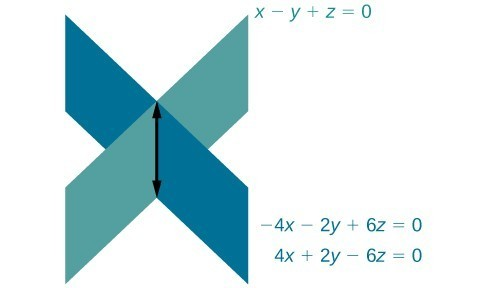## Inconsistent and Dependent Systems in Three Variables

Just as with systems of equations in two variables, we may come across an inconsistent system of equations in three variables, which means that it does not have a solution that satisfies all three equations. The equations could represent three parallel planes, two parallel planes and one intersecting plane, or three planes that intersect the other two but not at the same location. The process of elimination will result in a false statement, such as $3=7$ or some other contradiction.

### Example 4: Solving an Inconsistent System of Three Equations in Three Variables

Solve the following system.

$\begin{array}{ll}\text{ }x - 3y+z=4\hfill & \left(1\right)\hfill \\ \text{ }-x+2y - 5z=3\hfill & \left(2\right)\hfill \\ 5x - 13y+13z=8\hfill & \left(3\right)\hfill \end{array}$

### Solution

Looking at the coefficients of $x$, we can see that we can eliminate $x$ by adding equation (1) to equation (2).

$\frac{\begin{array}{l}\text{ }x - 3y+z=4\text{ }\left(1\right)\hfill \\ -x+2y - 5z=3\text{ }\left(2\right)\hfill \end{array}}{\text{ }-y - 4z=7\text{ }\left(4\right)}$

Next, we multiply equation (1) by $-5$ and add it to equation (3).

$\begin{array}−5x+15y−5z=−20 \hfill& \left(1\right)\text{ multiplied by }−5 \\ 5x−13y+13z=8 \hfill& \left(3\right) \\ \text{___________________} \\ 2y+8z=−12\hfill&\left(5\right) \end{array}$

Then, we multiply equation (4) by 2 and add it to equation (5).

$\begin{array}−2y−8z=14 \hfill& \left(4\right)\text{ multiplied by }2 \\ 2y+8z=−12 \hfill& \left(5\right) \\ \text{___________________} \\ 0=2\end{array}$

The final equation $0=2$ is a contradiction, so we conclude that the system of equations in inconsistent and, therefore, has no solution.

### Analysis of the Solution

In this system, each plane intersects the other two, but not at the same location. Therefore, the system is inconsistent.

### Try It 2

Solve the system of three equations in three variables.

$\begin{array}{l}\text{ }x+y+z=2\hfill \\ \text{ }y - 3z=1\hfill \\ 2x+y+5z=0\hfill \end{array}$

## Expressing the Solution of a System of Dependent Equations Containing Three Variables

We know from working with systems of equations in two variables that a dependent system of equations has an infinite number of solutions. The same is true for dependent systems of equations in three variables. An infinite number of solutions can result from several situations. The three planes could be the same, so that a solution to one equation will be the solution to the other two equations. All three equations could be different but they intersect on a line, which has infinite solutions. Or two of the equations could be the same and intersect the third on a line.

### Example 5: Finding the Solution to a Dependent System of Equations

Find the solution to the given system of three equations in three variables.

$\begin{array}{rr}\hfill \text{ }2x+y - 3z=0& \hfill \left(1\right)\\ \hfill 4x+2y - 6z=0& \hfill \left(2\right)\\ \hfill \text{ }x-y+z=0& \hfill \left(3\right)\end{array}$

### Solution

First, we can multiply equation (1) by $-2$ and add it to equation (2).

$\begin{array} −4x−2y+6z=0 \hfill& \text{equation }\left(1\right)\text{multiplied by }−2 \\ 4x+2y−6z=0\hfill&\left(2\right) \end{array}$

We do not need to proceed any further. The result we get is an identity, $0=0$, which tells us that this system has an infinite number of solutions. There are other ways to begin to solve this system, such as multiplying equation (3) by $-2$, and adding it to equation (1). We then perform the same steps as above and find the same result, $0=0$.

When a system is dependent, we can find general expressions for the solutions. Adding equations (1) and (3), we have

$\begin{array}2x+y−3z=0 \\ x−y+z=0 \\ \text{______________} \\ 3x−2z=0 \end{array}$

We then solve the resulting equation for $z$.

$\begin{array}{l}3x - 2z=0\hfill \\ \text{ }z=\frac{3}{2}x\hfill \end{array}$

We back-substitute the expression for $z$ into one of the equations and solve for $y$.

$\begin{array}{l}2x+y - 3\left(\frac{3}{2}x\right)=0\hfill \\ \text{ }2x+y-\frac{9}{2}x=0\hfill \\ \text{ }y=\frac{9}{2}x - 2x\hfill \\ \text{ }y=\frac{5}{2}x\hfill \end{array}$

So the general solution is $\left(x,\frac{5}{2}x,\frac{3}{2}x\right)$. In this solution, $x$ can be any real number. The values of $y$ and $z$ are dependent on the value selected for $x$.

### Analysis of the Solution

As shown in Figure 5, two of the planes are the same and they intersect the third plane on a line. The solution set is infinite, as all points along the intersection line will satisfy all three equations.Figure 5

### Does the generic solution to a dependent system always have to be written in terms of $x?$

No, you can write the generic solution in terms of any of the variables, but it is common to write it in terms of x and if needed $x$ and $y$.

### Try It 3

Solve the following system.

$\begin{array}{l}\text{ }x+y+z=7\hfill \\ 3x - 2y-z=4\hfill \\ x+6y+5z=24\hfill \end{array}$